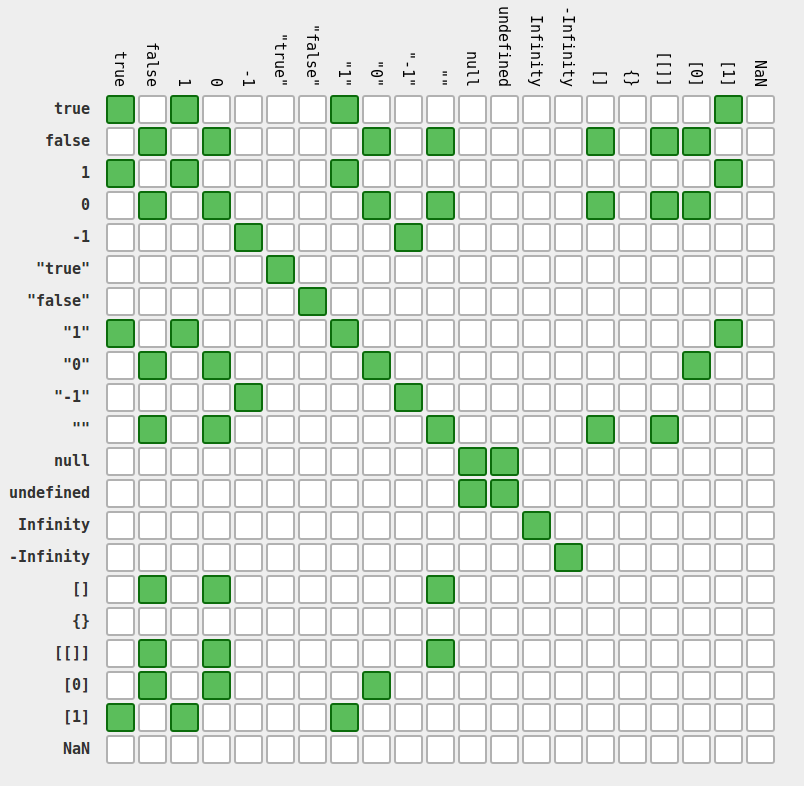### If (Boolean) equals true

Avoid comparing a Boolean value to `true` or `false`

``````if ((score >= 50) === true) {
console.log('pass');
}
``````

The above would be more succinctly stated as:

``````if (score >= 50) {
console.log('pass');
}
``````

In a similar vein, avoid assigning Boolean values by means of a ternary operator:

``````var pass = (score >= 50) ? true : false;
``````

Instead, this can be expressed more succinctly, like so:

``````var pass = (score >= 50);
``````

Note that when doing this, the operator precedence means that `score >= 50` does not need to be surrounded by parentheses, however, doing so makes the code easier to understand at first glance.

### Testing for `null` and `undefined`

Quite often you will find yourself testing whether a variable has been set. In Javascript, this means that you want to check if it has been set to either `null` or `undefined`.

A simple way of testing whether a variable is `null` or `undefined` could be simply:

``````var isNull = (myVar === null); //OK
var isUndefined = (myVar === undefined); //NOT OK
``````

The first statement is OK, because `null` is immutable. However, one of Javascript’s quirks is that `undefined` is, for some reason, mutable. This means that someone could assign a value to `undefined`, even a “truthy” one, thus impacting any other comparisons.

``````undefined = 2; //Do NOT do this - Javascript quirk demo only
var isUndefined = (myVar === undefined);
``````

In the example above, if the value of `myVar` was `2`, `isUndefined` will be equal to `true`, which is confusing, to say the least.

The correct way to test if a variable is `undefined`, is to use the `typeof` operator:

``````var isUndefined = (typeof myVar === 'undefined');
``````

However, often times, you do not really care if any variable is exactly `undefined` or `null`, and you want to do the same thing in either case.

Both of these values are special values which are considered “falsey”, meaning that they are loosely equal to `false` (more on strict and loose equality below).

Thus, a simplified test can be done instead:

``````var isFalsey = (!myVar);
``````

Note that `isFalsey` will be true when `myVar` is one of the following:

• `undefined`
• `null`
• any other values which are “falsey”, e.g. `0` or an empty string.

### Strict Equality

Most of the time, you want to know if the values stored in two variables are exactly the same. Sometimes, however, you simply want to know if they are roughly the same thing. In Javascript, there are two forms of equality comparison: strict equality and loose equality:

• `==` : loose equals
• `!=` : loose not equals
• `===` : strict equals
• `!==` : strict not equals

If your intent is to determine whether two variable are exactly the same, do not use `==` or `!=`. Use `===` or `!==` instead!

This is because the former (double equals) attempts to do type coercion before comparison, whereas the latter (triple equals) simply compares as-is.

For example, and empty string and `0` are not strictly equal, but they are indeed loosely equal. Javascript is full of little surprises like that - making the equality comparison which occurs with a type coercion is notoriously difficult to comprehend so it is best to avoid it!If you cannot memorise this chart easily, you are probably much better off using the triple equals form.